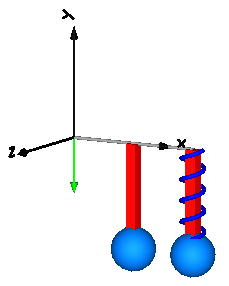# Spring-mass system

This example mirrors that of the modelica spring-mass system and demonstrates that we can model a spring-mass system in two different ways.

1. Using a prismatic joint and a 1-dimensional spring from the Translational submodule attached to the joint. The advantage of this approach is that the many elements from the Translational library can be easily used here and that this implementation is usually more efficient compared to when using 3-dimensional springs.
2. Using a 3-dimensional spring from the Multibody library.using Multibody
using ModelingToolkit
using Plots
using SymbolicIR
using OrdinaryDiffEq

t = Multibody.t
D = Differential(t)
world = Multibody.world

systems = @named begin
p1      = Prismatic(n = [0, -1, 0], s0 = 0.1, useAxisFlange = true)
spring1 = Translational.Spring(30, s_rel0 = 0.1)
spring2 = Multibody.Spring(c = 30, s_unstretched = 0.1)
body1   = Body(m = 1, r_cm = [0, 0, 0])
bar1    = FixedTranslation(r = [0.3, 0, 0])
bar2    = FixedTranslation(r = [0.3, 0, 0])
body2   = Body(m = 1, r_cm = [0, 0, 0])
p2      = Prismatic(n = [0, -1, 0], s0 = 0.1, useAxisFlange = true)
end

eqs = [
connect(body1.frame_a, p1.frame_b)
connect(world.frame_b, bar1.frame_a)
connect(bar1.frame_b, p1.frame_a)
connect(spring1.flange_b, p1.axis)
connect(bar1.frame_b, bar2.frame_a)
connect(bar2.frame_b, p2.frame_a)
connect(p2.frame_b, body2.frame_a)
connect(bar2.frame_b, spring2.frame_a)
connect(body2.frame_a, spring2.frame_b)
connect(spring1.flange_a, p1.support)
]

@named model = ODESystem(eqs, t, systems = [world; systems])
ssys = structural_simplify(IRSystem(model))
prob = ODEProblem(ssys,[
D(p1.s) => 0,
D(D(p1.s)) => 0,
D(p2.s) => 0,
D(D(p2.s)) => 0,
], (0, 10))

sol = solve(prob, Rodas4())
@assert SciMLBase.successful_retcode(sol)

plot(sol, idxs = [body1.r_0, body2.r_0])

The plot indicates that the two systems behave identically.